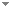Switch to: References

Replacing Causal Faithfulness with Algorithmic Independence of Conditionals

Minds and Machines 23 (2):227-249 (2013)

 Detecting Confounding in Multivariate Linear Models Via Spectral Analysis.Dominik Janzing & Bernhard Schölkopf - 2017 - Journal of Causal Inference 6 (1).We study a model where one target variable$Y$is correlated with a vector$\textbf{X}:=$of predictor variables being potential causes of$Y$. We describe a method that infers to what extent the statistical dependences between$\textbf{X}$and$Y$are due to the influence of$\textbf{X}$on$Y$and to what extent due to a hidden common cause of$\textbf{X}$and$Y$. The method relies on concentration of measure results for large dimensions$d$and an independence assumption stating that, in the absence of confounding, the vector of regression coefficients describing the influence of each$\textbf{X}$on$Y$typically has ‘generic orientation’ relative (...) Direct download (5 more)     Export citationBookmarkCausal Versions of Maximum Entropy and Principle of Insufficient Reason.Dominik Janzing - 2021 - Journal of Causal Inference 9 (1):285-301.The principle of insufficient reason assigns equal probabilities to each alternative of a random experiment whenever there is no reason to prefer one over the other. The maximum entropy principle generalizes PIR to the case where statistical information like expectations are given. It is known that both principles result in paradoxical probability updates for joint distributions of cause and effect. This is because constraints on the conditional P P\left result in changes of P P\left that assign higher probability to those (...)No categories Direct download     Export citationBookmarkMarkov Boundary Discovery with Ridge Regularized Linear Models.V. Strobl Eric & Visweswaran Shyam - 2015 - Journal of Causal Inference. Direct download (6 more)     Export citationBookmark